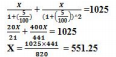Courses

Compound Interest - MCQ 4

20 Questions MCQ Test Quantitative Ability for SSC CHSL | Compound Interest - MCQ 4

Description
Attempt Compound Interest - MCQ 4 | 20 questions in 15 minutes | Mock test for Quant preparation | Free important questions MCQ to study Quantitative Ability for SSC CHSL for Quant Exam | Download free PDF with solutions
QUESTION: 1

What is the difference between the CI and SI on Rs.6500 at the rate of 4 pcpa in 2 yrs ?

Solution:

SI = 6500*4*2/100 = 520
CI = 6500*(104/100)*(104/100) = 7030.4 = 530.4
530.4 – 520 = 10.4

QUESTION: 2

The CI on a certain sum at 10% pa for 2yrs is Rs.6548. What is SI on the sum of money at 7%pa for 4 yrs(approx) ?

Solution:

CI = p [{1 + (10/100)}2 – 1] 6548 = p [(110/100)2 – 1] 6548 =p (121 – 100)/100
P = 654800/21 =31,181
SI = 31181*7*4/100 = 8731

QUESTION: 3

A sum of money amount to Rs.900 in 3 yrs and Rs.1080 in 4yrs at CI. What is the rate of CI pa ?

Solution:

(1080-900/900)*100
180*100/900 = 20%

QUESTION: 4

Find the CI on Rs.4500 at 4% pa for 2 yrs compounded half yearly(approx) ?

Solution:

CI = P [(1+(r/100) – 1] = 4500[(1+2/100)4 – 1
= 4500[(102*102*102*102/100*100*100*100) – 1] = 4500[1.082 – 1] = 4500*0.082 = 369

QUESTION: 5

In how many years will a sum of Rs.15625 at 8% pa compounded semi annually become Rs.17,576?

Solution:

15625*(1+(4/100))2n = 17576
15625*(104/100)2n = 17576
(26/25)2n = 17576/15625 = (26/27)3
2n = 3 N =3/2 = 1(1/2) yrs

QUESTION: 6

If 40% increase in an amount in 4 years at SI. What will be the CI of Rs.10,000 after 2 yrs at the same rate ?

Solution:

P = 100, SI = 40, T = 4
R = 100*40 / 100*4 = 10%pa
CI = 10,000(110/100)2 = 10000*11*11/100 = 12100
12100 – 10000 = 2100

QUESTION: 7

A sum of money is borrowed and paid in 2 annual instalments of Rs.642 each allowing 4% CI. The sum borrowed was

Solution:

P = [642/(104/100)] + [642/(104/100) 2)
= 642*(25/26) + 642 (25/26)2
= 617.3 + 593.6 = 1210.9 = 1211

QUESTION: 8

The least number of complete years in which a sum of money put out at 10% CI will be more than doubled is

Solution:

P(110/100)n > 2P
(11/10)n > 2P
1.1*1.1*1.1*1.1*1.1*1.1*1.1*1.1 = 2.14 > 2
N = 8

QUESTION: 9

A sum of money doubles itself at CI in 10yrs. In how many yrs will it become 4 times ?

Solution:

P(1+(R/100))10 = 2P
1+(R/100)10 = 2
(1+(R/100))n = 4 = 22
2*10 = 20yrs

QUESTION: 10

At what rate of CI pa will a sum of Rs.1000 becomes Rs.1040.4 in 2yrs ?

Solution:

1000*(1+(R/100))2 = 1040.4
(1+(R/100))2 = 1040.4/1000
(1+(R/100))2 = 10404/10000 = (102/100)2
R = 2%

QUESTION: 11

What annual payment will discharge a debt of 1025 due in 2 years at the rate of 5% compound interest?

Solution:QUESTION: 12

In what time will Rs. 64,000 amount to Rs.68921 at 5% per annum interest being compounded half yearly?

Solution:

R = 2.5%, A = 68921 , P = 64000 and t= 2n
A/P = [1+(R/100)]2n
68921/64000 = [1+(2.5/100)]2n
(41/40)3 = (41/40)2n
2n = 3
n=3/2 =1(1/2) years

QUESTION: 13

If the difference between the CI and SI on a sum of money at 5% per annum for 2years is Rs.16.Find the Simple Interest ?

Solution:

16 =P*(5/100)2
P = 6400
SI =(6400*5*2)/100 =640

QUESTION: 14

The difference between the SI and CI on Rs.5000 at 10%per annum for 2 year is

Solution:

d = p (r/100)2
= 5000 (10/100)2
d = 50

QUESTION: 15

The difference SI and CI on Rs.1000 for 1 year at 10%per annum reckoned Half yearly is

Solution:

SI = (1000/100 =100
CI = [1000(1+(5/100))2] – 1000 = 102.5
D = 102.5 – 100 = 2.5

QUESTION: 16

Compound interest on a certain sum of money at 20% per annum for 2 years is Rs.5984.What is the SI on the same money at 9% per annum for 6 years ?

Solution:

5984 = p [(1+(20/100)2) -1] P = (5984*25)/11 = 13600
SI = (13600*9*6)/100 = 7344

QUESTION: 17

The difference between CI and SI on an amount Rs. 15000 for 2 year is Rs.96.What is the rate of interest per annum ?

Solution:
QUESTION: 18

The effective annual rate of interest corresponding to the nominal rate of 4% per annum payable half yearly is

Solution:

Let p = 100
CI = [100+(1+[2/100]2)] = 100 × (102/100)× (102/100)
= 104.04
Effective rate = 104.04 – 100 = 4.04%

QUESTION: 19

Rohit borrowed Rs. 1200 at 12% PA .He repaid Rs. 500 at the end of 1 year. What is the amount required to pay at the end of 2nd year to discharge his loan which was calculated in CI

Solution:

CI at the end of 1st year = 1200 *(1+(12 /100)) = 1344
CI = 1344-1200 = 144
500 paid then remaining amount = 1344 – 500 = 844
At the end of 2nd year
844*[(1+(12/100)] =945.28

QUESTION: 20

A sum of money invested at CI to Rs.800 in 3 years and to Rs.840 in 4 years.Find rate of interest PA ?

Solution:

CI for 3 yr = 800
CI for 4 yr = 840
CI for 1 yr = 40
R = (100 × 40)/(800×1) = 10/2 = 5%Use Code STAYHOME200 and get INR 200 additional OFF Use Coupon Code

Track your progress, build streaks, highlight & save important lessons and more!

Similar ContentRelated tests